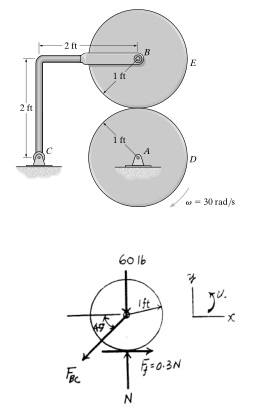# Direction of friction between disks

## Homework StatementDisk D turns with a constant clockwise angular
velocity of 30 . Disk E has a weight of 60 lb and is
initially at rest when it is brought into contact with D.
Determine the time required for disk E to attain the same
angular velocity as disk D. The coefficient of kinetic
friction between the two disks is 0.3 . Neglect the
weight of bar BC.

2. Relevant equation
Summation of forces and moments.

## The Attempt at a Solution

I am confused with the direction of friction. In my calculations, the friction points to the left because the velocity where the disk meets points to the right; which resulted to a wrong answer. However, the correct free body diagram is shown above.

Bystander
Homework Helper
Gold Member
the friction points to the left
That is the force that "what" exerts on "what else?" And what is the reaction force to that?

So when I isolate disk D, the friction points to the left to counter the clockwise motion, and when I isolate disk E, it now points to the right. Is this right?

Bystander
•